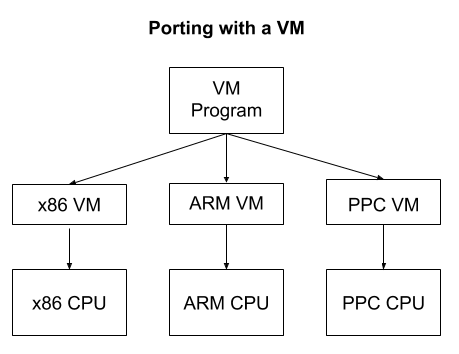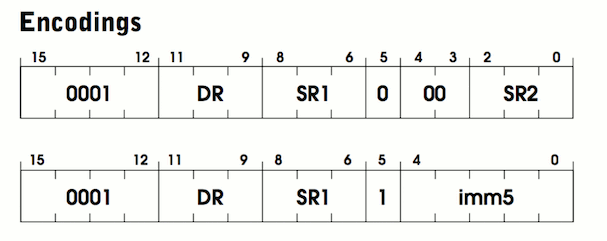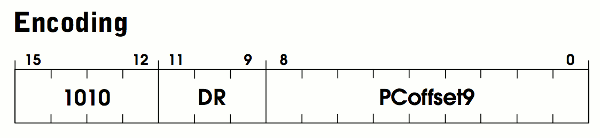# [译] 400 行 C 代码实现一个虚拟机（2018）

Published at 2019-10-16 | Last Update 2021-02-22

# 1. 引言

## 什么是虚拟机？Java Virtual Machine (JVM) 就是一个非常成功的例子。JVM 本身是一个中等大小、程序员完全能够看懂的程序，因此很 容易将它移植到包括手机在内的上千种设备上。只要在设备上实现了 JVM，接下来任何 Java、Kotlin 或 Clojure 程序都无需任何修改就可以直接运行在这个设备上。唯一的开销 来自虚拟机自身以及机器之上的 进一步抽象。 大部分情况下，这完全是可以接受的。

# 2. LC-3 架构

## 2.1 内存

LC-3 有 65,536 个内存位置（16 bit 无符号整形能寻址的最大值），每个位置可以存储一 个 16-bit 的值。这意味着它总共可以存储 128KB 数据（64K * 2 Byte），比我们平时接触 的计算机内存小多了！在我们的程序中，这个内存会以简单数组的形式存放数据：

``````/* 65536 locations */
uint16_t memory[UINT16_MAX];
``````

## 2.2 寄存器

LC-3 总共有 10 个寄存器，每个都是 16 比特。其中大部分都是通用目的寄存器，少数几 个用于特定目的。

• 8 个通用目的寄存器（R0-R7）
• 1 个程序计数器（program counter, PC）寄存器
• 1 个条件标志位（condition flags，COND）寄存器

``````enum {
R_R0 = 0,
R_R1,
R_R2,
R_R3,
R_R4,
R_R5,
R_R6,
R_R7,
R_PC, /* program counter */
R_COND,
R_COUNT
};
``````

``````uint16_t reg[R_COUNT];
``````

## 2.3 指令集

1. 操作码（opcode）：表示任务的类型
2. 执行任务所需的参数

``````enum {
OP_BR = 0, /* branch */
OP_ST,     /* store */
OP_JSR,    /* jump register */
OP_AND,    /* bitwise and */
OP_STR,    /* store register */
OP_RTI,    /* unused */
OP_NOT,    /* bitwise not */
OP_STI,    /* store indirect */
OP_JMP,    /* jump */
OP_RES,    /* reserved (unused) */
OP_TRAP    /* execute trap */
};
``````

## 2.4 条件标志位

`R_COND` 寄存器存储条件标记，其中记录了最近一次计算的执行结果。 这使得程序可以完成诸如 `if (x > 0) { ... }` 之类的逻辑条件。

``````enum {
FL_POS = 1 << 0, /* P */
FL_ZRO = 1 << 1, /* Z */
FL_NEG = 1 << 2, /* N */
};
``````

# 3. 汇编示例

``````.ORIG x3000                        ; this is the address in memory where the program will be loaded
LEA R0, HELLO_STR                  ; load the address of the HELLO_STR string into R0
PUTs                               ; output the string pointed to by R0 to the console
HALT                               ; halt the program
HELLO_STR .STRINGZ "Hello World!"  ; store this string here in the program
.END                               ; mark the end of the file
```````.ORIG``.STRINGZ` 看起来像是指令，但其实不是，它们称为汇编制导命令 （assembler directives），可以生成一段代码或数据。例如，`.STRINGZ` 会在它所在的 位置插入一段字符串。

``````AND R0, R0, 0                      ; clear R0
LOOP                               ; label at the top of our loop
ADD R0, R0, 1                      ; add 1 to R0 and store back in R0
ADD R1, R0, -10                    ; subtract 10 from R0 and store back in R1
BRn LOOP                           ; go back to LOOP if the result was negative
... ; R0 is now 10!
``````

# 4. 执行程序

“The view that machines cannot give rise to surprises is due, I believe, to a fallacy to which philosophers and mathematicians are particularly subject. This is the assumption that as soon as a fact is presented to a mind all consequences of that fact spring into the mind simultaneously with it. It is a very useful assumption under many circumstances, but one too easily forgets that it is false.” — Alan M. Turing

## 过程（Procedure）

1. 从 PC 寄存器指向的内存地址中加载一条指令
2. 递增 PC 寄存器
3. 查看指令中的 opcode 字段，判断指令类型
4. 根据指令类型和指令中所带的参数执行该指令
5. 跳转到步骤 1

``````int main(int argc, const char* argv[]) {
{Setup, 12}

/* set the PC to starting position */
enum { PC_START = 0x3000 }; /* 0x3000 is the default */
reg[R_PC] = PC_START;

int running = 1;
while (running) {
uint16_t instr = mem_read(reg[R_PC]++); /* FETCH */
uint16_t op = instr >> 12;

switch (op) {
case OP_AND: {AND, 7} break;
case OP_NOT: {NOT, 7} break;
case OP_BR: {BR, 7} break;
case OP_JMP: {JMP, 7} break;
case OP_JSR: {JSR, 7} break;
case OP_LD: {LD, 7} break;
case OP_LDI: {LDI, 6} break;
case OP_LDR: {LDR, 7} break;
case OP_LEA: {LEA, 7} break;
case OP_ST: {ST, 7} break;
case OP_STI: {STI, 7} break;
case OP_STR: {STR, 7} break;
case OP_TRAP: {TRAP, 8} break;
case OP_RES:
case OP_RTI:
default:
break;
}
}
{Shutdown, 12}
}
``````

# 5. 指令实现• 两者都是以 `0001` 这 4 个比特开始的，这是 OP_ADD 的操作码（opcode）
• 后面 3 个比特名为 `DR`（destination register），即目的寄存器，相加的结果会放到 这里
• 再后面 3 个比特是 `SR1`，这个寄存器存放了第一个将要相加的数字

``````ADD R2 R0 R1 ; add the contents of R0 to R1 and store in R2.
``````

``````ADD R0 R0 1 ; add 1 to R0 and store back in R0
``````

If bit  is 0, the second source operand is obtained from SR2. If bit  is 1, the second source operand is obtained by sign-extending the imm5 field to 16 bits. In both cases, the second source operand is added to the contents of SR1 and the result stored in DR. (Pg. 526)

``````uint16_t sign_extend(uint16_t x, int bit_count) {
if ((x >> (bit_count - 1)) & 1) {
x |= (0xFFFF << bit_count);
}
return x;
}
``````

The condition codes are set, based on whether the result is negative, zero, or positive. (Pg. 526)

``````void update_flags(uint16_t r) {
if (reg[r] == 0) {
reg[R_COND] = FL_ZRO;
}
else if (reg[r] >> 15) { /* a 1 in the left-most bit indicates negative */
reg[R_COND] = FL_NEG;
} else {
reg[R_COND] = FL_POS;
}
}
``````

``````{
uint16_t r0 = (instr >> 9) & 0x7; /* destination register (DR) */
uint16_t r1 = (instr >> 6) & 0x7; /* first operand (SR1) */
uint16_t imm_flag = (instr >> 5) & 0x1; /* whether we are in immediate mode */

if (imm_flag) {
uint16_t imm5 = sign_extend(instr & 0x1F, 5);
reg[r0] = reg[r1] + imm5;
} else {
uint16_t r2 = instr & 0x7;
reg[r0] = reg[r1] + reg[r2];
}

update_flags(r0);
}
``````

• 在寄存器模式中，第二个值存储在某个寄存器中
• 在立即模式中，第二个值存储在指令最右边的 5 个比特中
• 短于 16 比特的值需要执行有符号扩展
• 每次指令修改了寄存器后，都需要更新条件标志位（condition flags）

## 5.2 LDI

LDI 是 load indirect 的缩写，用于从内存加载一个值到寄存器，规范见 532 页。 LDI 的二进制格式如下：An address is computed by sign-extending bits [8:0] to 16 bits and adding this value to the incremented PC. What is stored in memory at this address is the address of the data to be loaded into DR. (Pg. 532)

``````// the value of far_data is an address
// of course far_data itself (the location in memory containing the address) has an address
char* far_data = "apple";

// In memory it may be layed out like this:

// 0x123:  far_data = 0x456
// ...
// 0x456:  string   = 'a'

// if PC was at 0x100
// LDI R0 0x023
// would load 'a' into R0
``````

The condition codes are set based on whether the value loaded is negative, zero, or positive. (Pg. 532)

``````{
uint16_t r0 = (instr >> 9) & 0x7; /* destination register (DR) */
uint16_t pc_offset = sign_extend(instr & 0x1ff, 9); /* PCoffset 9*/

/* add pc_offset to the current PC, look at that memory location to get the final address */
update_flags(r0);
}
``````

# 6. 全部指令的参考实现

## 6.1 RTI & RES

``````abort();
``````

## 6.2 Bitwise and（按位与）

``````{
uint16_t r0 = (instr >> 9) & 0x7;
uint16_t r1 = (instr >> 6) & 0x7;
uint16_t imm_flag = (instr >> 5) & 0x1;

if (imm_flag) {
uint16_t imm5 = sign_extend(instr & 0x1F, 5);
reg[r0] = reg[r1] & imm5;
} else {
uint16_t r2 = instr & 0x7;
reg[r0] = reg[r1] & reg[r2];
}
update_flags(r0);
}
``````

## 6.3 Bitwise not（按位非）

``````{
uint16_t r0 = (instr >> 9) & 0x7;
uint16_t r1 = (instr >> 6) & 0x7;

reg[r0] = ~reg[r1];
update_flags(r0);
}
``````

## 6.4 Branch（条件分支）

``````{
uint16_t pc_offset = sign_extend((instr) & 0x1ff, 9);
uint16_t cond_flag = (instr >> 9) & 0x7;
if (cond_flag & reg[R_COND]) {
reg[R_PC] += pc_offset;
}
}
``````

## 6.5 Jump（跳转）

RET 在规范中作为一个单独的指令列出，因为在汇编中它是一个独立的关键字。但是，RET 本质上是 JMP 的一个特殊情况。当 R1 为 7 时会执行 RET。

``````{
/* Also handles RET */
uint16_t r1 = (instr >> 6) & 0x7;
reg[R_PC] = reg[r1];
}
``````

## 6.6 Jump Register（跳转寄存器）

``````{
uint16_t r1 = (instr >> 6) & 0x7;
uint16_t long_pc_offset = sign_extend(instr & 0x7ff, 11);
uint16_t long_flag = (instr >> 11) & 1;

reg[R_R7] = reg[R_PC];
if (long_flag) {
reg[R_PC] += long_pc_offset;  /* JSR */
} else {
reg[R_PC] = reg[r1]; /* JSRR */
}
break;
}
``````

``````{
uint16_t r0 = (instr >> 9) & 0x7;
uint16_t pc_offset = sign_extend(instr & 0x1ff, 9);
update_flags(r0);
}
``````

``````{
uint16_t r0 = (instr >> 9) & 0x7;
uint16_t r1 = (instr >> 6) & 0x7;
uint16_t offset = sign_extend(instr & 0x3F, 6);
update_flags(r0);
}
``````

``````{
uint16_t r0 = (instr >> 9) & 0x7;
uint16_t pc_offset = sign_extend(instr & 0x1ff, 9);
reg[r0] = reg[R_PC] + pc_offset;
update_flags(r0);
}
``````

## 6.10 Store（存储）

``````{
uint16_t r0 = (instr >> 9) & 0x7;
uint16_t pc_offset = sign_extend(instr & 0x1ff, 9);
mem_write(reg[R_PC] + pc_offset, reg[r0]);
}
``````

## 6.11 Store Indirect（间接存储）

``````{
uint16_t r0 = (instr >> 9) & 0x7;
uint16_t pc_offset = sign_extend(instr & 0x1ff, 9);
}
``````

## 6.12 Store Register（存储寄存器）

``````{
uint16_t r0 = (instr >> 9) & 0x7;
uint16_t r1 = (instr >> 6) & 0x7;
uint16_t offset = sign_extend(instr & 0x3F, 6);
mem_write(reg[r1] + offset, reg[r0]);
}
``````

# 7. Trap Routines（中断陷入例程）

LC-3 提供了几个预定于的函数（过程），用于执行常规任务以及与 I/O 设备交换， 例如，用于从键盘接收输入的函数，在控制台上显示字符串的函数。这些都称为 trap routines，你可以将它们当做操作系统或者是 LC-3 的 API。 每个 trap routine 都有一个对应的 trap code（中断号）。要执行一次捕获， 需要用相应的 trap code 执行 `TRAP` 指令。``````enum {
TRAP_GETC = 0x20,  /* get character from keyboard, not echoed onto the terminal */
TRAP_OUT = 0x21,   /* output a character */
TRAP_PUTS = 0x22,  /* output a word string */
TRAP_IN = 0x23,    /* get character from keyboard, echoed onto the terminal */
TRAP_PUTSP = 0x24, /* output a byte string */
TRAP_HALT = 0x25   /* halt the program */
};
``````

TRAP 处理逻辑：

``````switch (instr & 0xFF) {
case TRAP_GETC: {TRAP GETC, 9} break;
case TRAP_OUT: {TRAP OUT, 9} break;
case TRAP_PUTS: {TRAP PUTS, 8} break;
case TRAP_IN: {TRAP IN, 9} break;
case TRAP_PUTSP: {TRAP PUTSP, 9} break;
case TRAP_HALT: {TRAP HALT, 9} break;
}
``````

## 7.1 PUTS

`PUT` trap code 用于输出一个以空字符结尾的字符串（和 C 中的 `printf` 类似）。规 范见 543 页。

Write a string of ASCII characters to the console display. The characters are contained in consecutive memory locations, one character per memory location, starting with the address specified in R0. Writing terminates with the occurrence of x0000 in a memory location. (Pg. 543)

``````{
/* one char per word */
uint16_t* c = memory + reg[R_R0];
while (*c) {
putc((char)*c, stdout);
++c;
}
fflush(stdout);
}
``````

# 8. Trap Routine 参考实现

## 8.1 输入单个字符（Input Character）

``````/* read a single ASCII char */
reg[R_R0] = (uint16_t)getchar();
``````

## 8.2 输出单个字符（Output Character）

``````putc((char)reg[R_R0], stdout);
fflush(stdout);
``````

## 8.3 打印输入单个字符提示（Prompt for Input Character）

``````printf("Enter a character: ");
char c = getchar();
putc(c, stdout);
reg[R_R0] = (uint16_t)c;
``````

## 8.4 输出字符串（Output String）

``````{
/* one char per byte (two bytes per word) here we need to swap back to
big endian format */
uint16_t* c = memory + reg[R_R0];
while (*c) {
char char1 = (*c) & 0xFF;
putc(char1, stdout);
char char2 = (*c) >> 8;
if (char2) putc(char2, stdout);
++c;
}
fflush(stdout);
}
``````

## 8.5 暂停程序执行（Halt Program）

``````puts("HALT");
fflush(stdout);
running = 0;
``````

# 9. 加载程序

``````void read_image_file(FILE* file) {
uint16_t origin; /* the origin tells us where in memory to place the image */
origin = swap16(origin);

/* we know the maximum file size so we only need one fread */
uint16_t max_read = UINT16_MAX - origin;
uint16_t* p = memory + origin;

/* swap to little endian */
*p = swap16(*p);
++p;
}
}
``````

``````uint16_t swap16(uint16_t x) {
return (x << 8) | (x >> 8);
}
``````

``````int read_image(const char* image_path) {
FILE* file = fopen(image_path, "rb");
if (!file) { return 0; };
fclose(file);
return 1;
}
``````

## 10. 内存映射寄存器（Memory Mapped Registers）

LC-3 有两个内存映射寄存器需要实现，分别是：

• `KBSR`：键盘状态寄存器（keyboard status register），表示是否有键按下
• `KBDR`：键盘数据寄存器（keyboard data register），表示哪个键按下了

``````enum {
MR_KBSR = 0xFE00, /* keyboard status */
MR_KBDR = 0xFE02  /* keyboard data */
};
``````

``````void mem_write(uint16_t address, uint16_t val) {
}

{
if (check_key()) {
memory[MR_KBSR] = (1 << 15);
memory[MR_KBDR] = getchar();
} else {
memory[MR_KBSR] = 0;
}
}
}
``````

## 11. 平台相关的细节

``````uint16_t check_key() {

struct timeval timeout;
timeout.tv_sec = 0;
timeout.tv_usec = 0;
return select(1, &readfds, NULL, NULL, &timeout) != 0;
}
``````

``````struct termios original_tio;

void disable_input_buffering() {
tcgetattr(STDIN_FILENO, &original_tio);
struct termios new_tio = original_tio;
new_tio.c_lflag &= ~ICANON & ~ECHO;
tcsetattr(STDIN_FILENO, TCSANOW, &new_tio);
}

void restore_input_buffering() {
tcsetattr(STDIN_FILENO, TCSANOW, &original_tio);
}
``````

``````void handle_interrupt(int signal) {
restore_input_buffering();
printf("\n");
exit(-2);
}
``````
``````signal(SIGINT, handle_interrupt);
disable_input_buffering();
``````

# 12. 运行虚拟机

``````Play 2048!
{2048 Example 13}
Control the game using WASD keys.
Are you on an ANSI terminal (y/n)? y
+--------------------------+
|                          |
|                          |
|                          |
|                     2    |
|                          |
|   2                      |
|                          |
|                          |
|                          |
+--------------------------+
``````

# 13. C++ 实现（可选）

C++ 有强大的编译时泛型（compile-time generics）机制，可以帮我们自动生成部分指令 的实现代码。这里的基本思想是重用每个指令的公共部分。例如，好几条指令都用到了间接 寻址或有符号扩展然后加到当前寄存器的功能。模板如下：

``````{Instruction C++ 14}
template <unsigned op>
void ins(uint16_t instr) {
uint16_t r0, r1, r2, imm5, imm_flag;
uint16_t pc_plus_off, base_plus_off;

uint16_t opbit = (1 << op);
if (0x4EEE & opbit) { r0 = (instr >> 9) & 0x7; }
if (0x12E3 & opbit) { r1 = (instr >> 6) & 0x7; }
if (0x0022 & opbit) {
r2 = instr & 0x7;
imm_flag = (instr >> 5) & 0x1;
imm5 = sign_extend((instr) & 0x1F, 5);
}
if (0x00C0 & opbit) {   // Base + offset
base_plus_off = reg[r1] + sign_extend(instr & 0x3f, 6);
}
if (0x4C0D & opbit) { // Indirect address
pc_plus_off = reg[R_PC] + sign_extend(instr & 0x1ff, 9);
}
if (0x0001 & opbit) {
// BR
uint16_t cond = (instr >> 9) & 0x7;
if (cond & reg[R_COND]) { reg[R_PC] = pc_plus_off; }
}
if (0x0002 & opbit) {  // ADD
if (imm_flag) {
reg[r0] = reg[r1] + imm5;
} else {
reg[r0] = reg[r1] + reg[r2];
}
}
if (0x0020 & opbit) {  // AND
if (imm_flag) {
reg[r0] = reg[r1] & imm5;
} else {
reg[r0] = reg[r1] & reg[r2];
}
}
if (0x0200 & opbit) { reg[r0] = ~reg[r1]; } // NOT
if (0x1000 & opbit) { reg[R_PC] = reg[r1]; } // JMP
if (0x0010 & opbit) {  // JSR
uint16_t long_flag = (instr >> 11) & 1;
pc_plus_off = reg[R_PC] +  sign_extend(instr & 0x7ff, 11);
reg[R_R7] = reg[R_PC];
if (long_flag) {
reg[R_PC] = pc_plus_off;
} else {
reg[R_PC] = reg[r1];
}
}

if (0x0004 & opbit) { reg[r0] = mem_read(pc_plus_off); } // LD
if (0x0040 & opbit) { reg[r0] = mem_read(base_plus_off); }  // LDR
if (0x4000 & opbit) { reg[r0] = pc_plus_off; } // LEA
if (0x0008 & opbit) { mem_write(pc_plus_off, reg[r0]); } // ST
if (0x0800 & opbit) { mem_write(mem_read(pc_plus_off), reg[r0]); } // STI
if (0x0080 & opbit) { mem_write(base_plus_off, reg[r0]); } // STR
if (0x8000 & opbit) {  // TRAP
{TRAP, 8}
}
//if (0x0100 & opbit) { } // RTI
if (0x4666 & opbit) { update_flags(r0); }
}
{Op Table 14}
static void (*op_table)(uint16_t) = {
ins<0>, ins<1>, ins<2>, ins<3>,
ins<4>, ins<5>, ins<6>, ins<7>,
NULL, ins<9>, ins<10>, ins<11>,
ins<12>, NULL, ins<14>, ins<15>
};
``````

• C
• C++
• Common Lisp
• Go